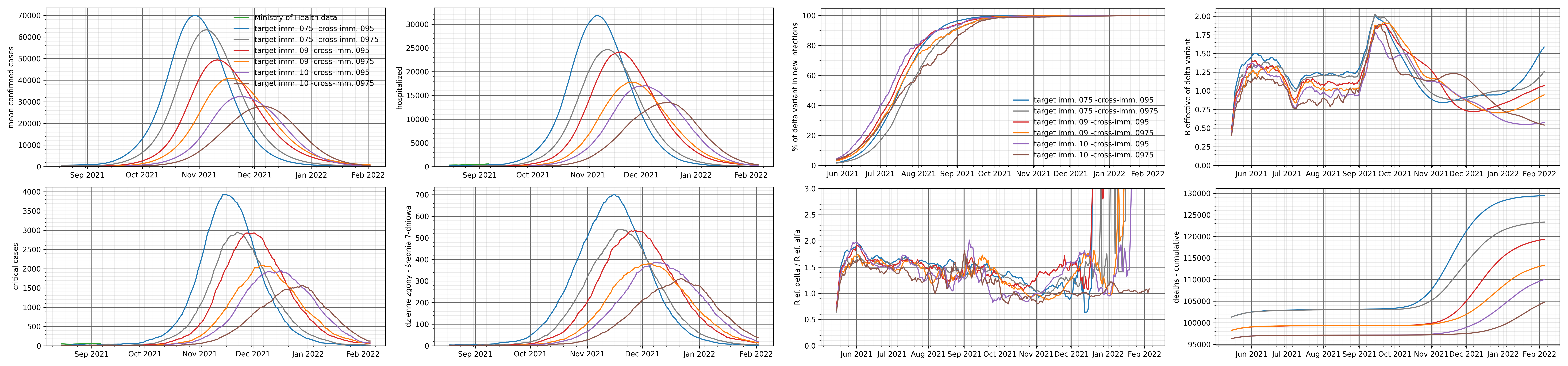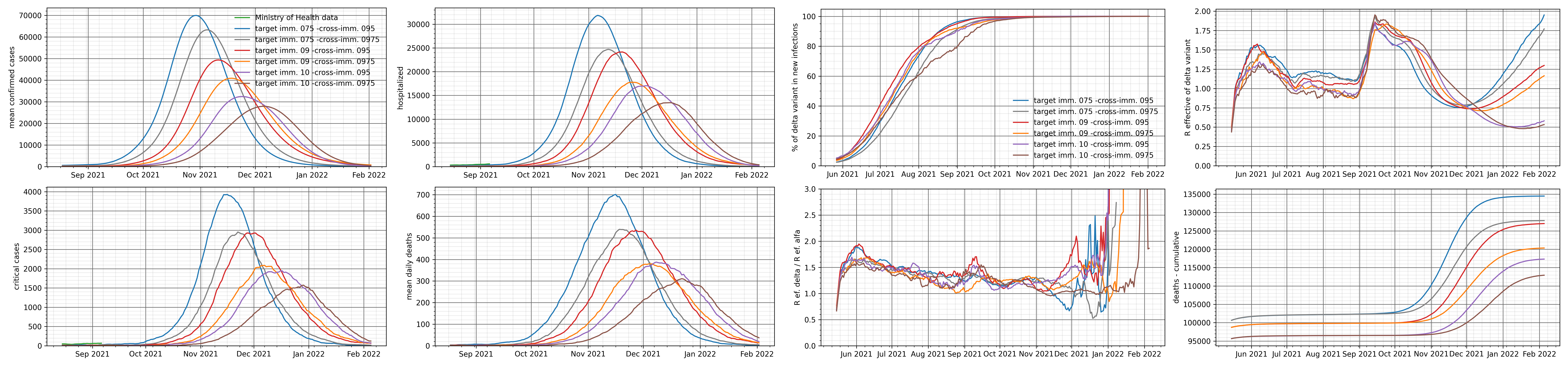# Variant prognosis for cross-resistance and reinfection

We present a variant forecast for the spreading of the epidemic in autumn 2021. This is a test forecast, taking into account the risk of reinfection and partial cross-resistance between the Alpha and Delta variants.

• The forecast variants assume two levels of population immunization (lower and higher) – the percentage of convalescents in the population on June 1, 2021 is approx. 45% or 57%, respectively.

• The forecasts take into account all historically introduced restrictions and easing, including the holiday period (school closings and increased travel) and assume administrative restrictions and the intensity of social contacts in the post-holiday period, analogous to September 2020.

• We assume the participation of the Delta variant in new infections on June 1 at the level of 6%.

• Vaccinations are implemented according to data provided by ECDC and take into account vaccinations for which the first dose was administered until August 14, 2021.

Main assumptions about the risk of reinfection
We assume that the level of immunity, understood as the percentage reduction in the daily probability of being infected for the second time with the same variant, is 100% for the first month after recovery and then slowly decreases linearly at a rate of 5% of the initial value per month.

We assume that the level of cross-resistance when exposed to the Delta variant after an outbreak of the wild or Alpha variant is initially 87.5%, 95% or 97.5%. This cross-resistance persists for one month after recovery and then slowly declines linearly at a rate of 5% of the starting value per month to an arbitrarily defined level.

High immunization: